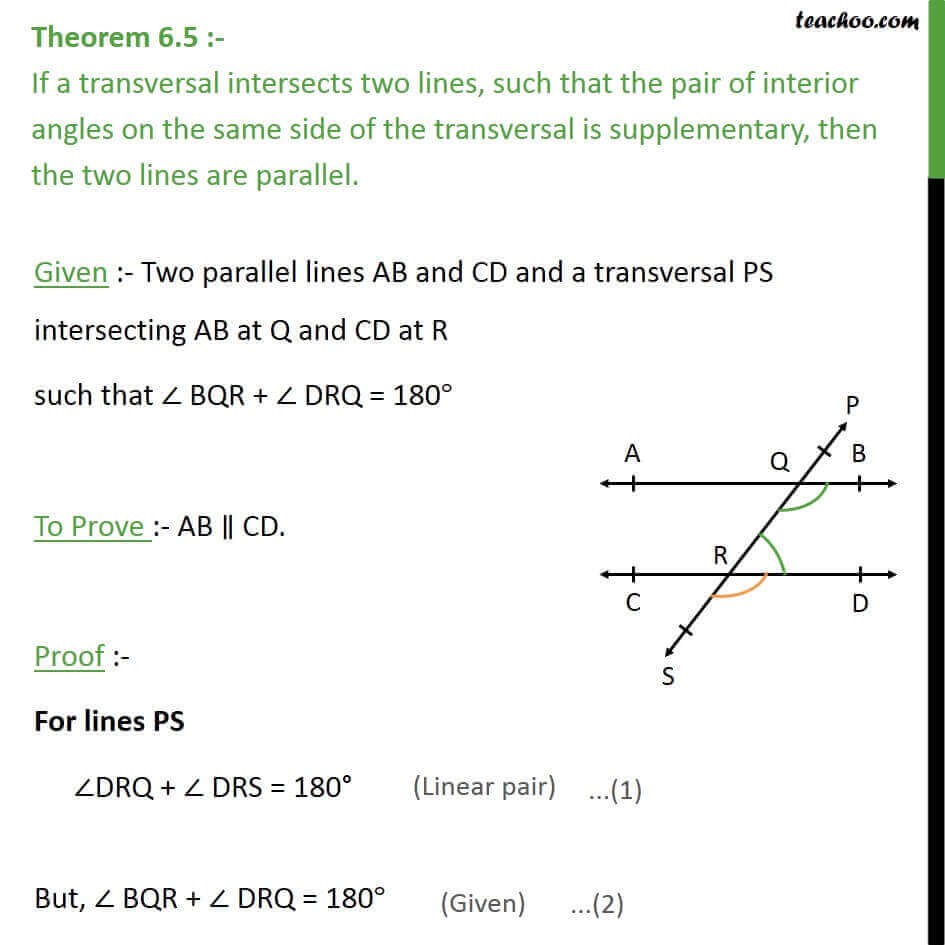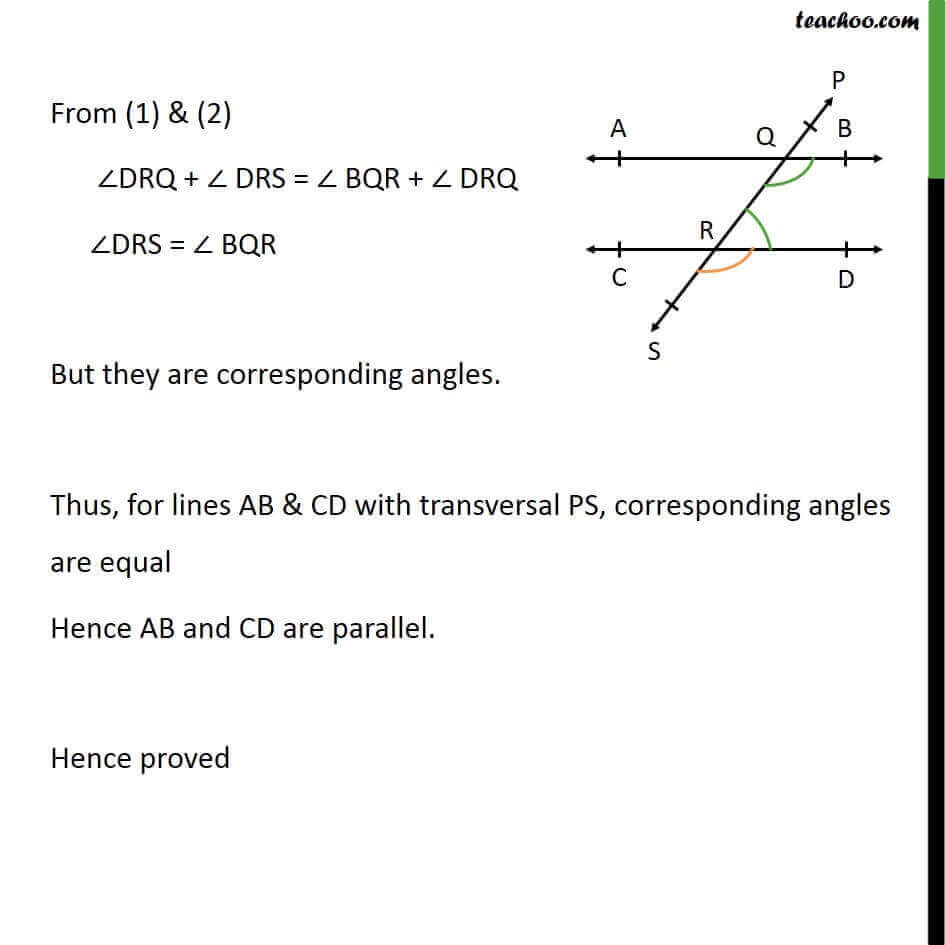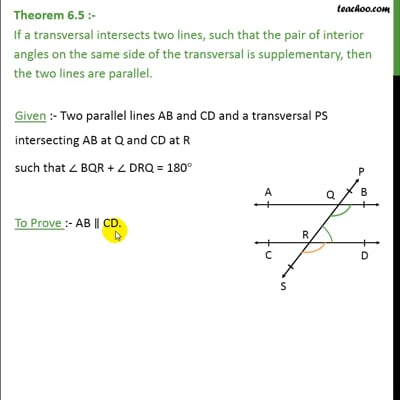Theorems

Chapter 6 Class 9 Lines and Angles (Term 1)
Serial order wiseThis video is only available for Teachoo black users

### Transcript

Theorem 6.5 :- If a transversal intersects two lines, such that the pair of interior angles on the same side of the transversal is supplementary, then the two lines are parallel. Given :- Two parallel lines AB and CD and a transversal PS intersecting AB at Q and CD at R such that BQR + DRQ = 180 To Prove :- AB CD. Proof :- For lines PS DRQ + DRS = 180 But, BQR + DRQ = 180 From (1) & (2) DRQ + DRS = BQR + DRQ DRS = BQR But they are corresponding angles. Thus, for lines AB & CD with transversal PS, corresponding angles are equal Hence AB and CD are parallel. Hence proved